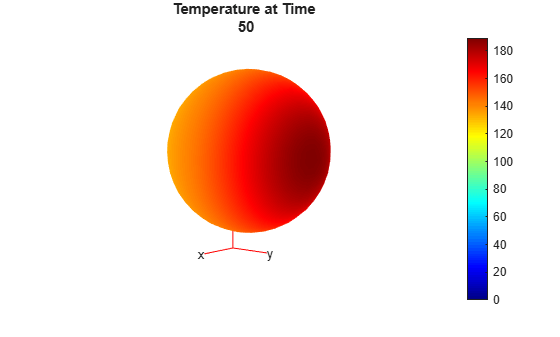# Heat Conduction in Multidomain Geometry with Nonuniform Heat Flux

This example shows how to perform a 3-D transient heat conduction analysis of a hollow sphere made of three different layers of material.

The sphere is subject to a nonuniform external heat flux.

The physical properties and geometry of this problem are described in Singh, Jain, and Rizwan-uddin (see Reference), which also has an analytical solution for this problem. The inner face of the sphere has a temperature of zero at all times. The outer hemisphere with positive $y$ value has a nonuniform heat flux defined by

`${q}_{outer}={\theta }^{2}\left(\pi -\theta {\right)}^{2}{\varphi }^{2}\left(\pi -\varphi {\right)}^{2}$`

`$0\le \theta \le \pi ,0\le \varphi \le \pi .$`

$\theta$ and $\varphi$ are azimuthal and elevation angles of points in the sphere. Initially, the temperature at all points in the sphere is zero.

Create a thermal model for transient thermal analysis.

`thermalmodel = createpde('thermal','transient');`

Create a multilayered sphere using the `multisphere` function. Assign the resulting geometry to the thermal model. The sphere has three layers of material with a hollow inner core.

```gm = multisphere([1,2,4,6],'Void',[true,false,false,false]); thermalmodel.Geometry = gm;```

Plot the geometry and show the cell labels and face labels. Use a `FaceAlpha` of 0.25 so that labels of the interior layers are visible.

```figure('Position',[10,10,800,400]); subplot(1,2,1) pdegplot(thermalmodel,'FaceAlpha',0.25,'CellLabel','on') title('Geometry with Cell Labels') subplot(1,2,2) pdegplot(thermalmodel,'FaceAlpha',0.25,'FaceLabel','on') title('Geometry with Face Labels')```Generate a mesh for the geometry. Choose a mesh size that is coarse enough to speed the solution, but fine enough to represent the geometry reasonably accurately.

`generateMesh(thermalmodel,'Hmax',1);`

Specify the thermal conductivity, mass density, and specific heat for each layer of the sphere. The material properties are dimensionless values, not given by realistic material properties.

```thermalProperties(thermalmodel,'Cell',1,'ThermalConductivity',1, ... 'MassDensity',1, ... 'SpecificHeat',1); thermalProperties(thermalmodel,'Cell',2,'ThermalConductivity',2, ... 'MassDensity',1, ... 'SpecificHeat',0.5); thermalProperties(thermalmodel,'Cell',3,'ThermalConductivity',4, ... 'MassDensity',1, ... 'SpecificHeat',4/9);```

Specify boundary conditions. The innermost face has a temperature of zero at all times.

`thermalBC(thermalmodel,'Face',1,'Temperature',0);`

The outer surface of the sphere has an external heat flux. Use the functional form of thermal boundary conditions to define the heat flux.

`function Qflux = externalHeatFlux(region,~)`

` [phi,theta,~] = cart2sph(region.x,region.y,region.z);`

` theta = pi/2 - theta; % transform to 0 <= theta <= pi`

` ids = phi > 0;`

` Qflux = zeros(size(region.x));`

` Qflux(ids) = theta(ids).^2.*(pi - theta(ids)).^2.*phi(ids).^2.*(pi - phi(ids)).^2;`

`end`

Plot the flux on the surface.

```[phi,theta,r] = meshgrid(linspace(0,2*pi),linspace(-pi/2,pi/2),6); [x,y,z] = sph2cart(phi,theta,r); region.x = x; region.y = y; region.z = z; flux = externalHeatFlux(region,[]); figure surf(x,y,z,flux,'LineStyle','none') axis equal view(130,10) colorbar xlabel 'x' ylabel 'y' zlabel 'z' title('External Flux')```Include this boundary condition in the model.

`thermalBC(thermalmodel,'Face',4,'HeatFlux',@externalHeatFlux,'Vectorized','on');`

Define the initial temperature to be zero at all points.

`thermalIC(thermalmodel,0);`

Define a time-step vector and solve the transient thermal problem.

```tlist = [0,2,5:5:50]; R = solve(thermalmodel,tlist);```

To plot contours at several times, with the contour levels being the same for all plots, determine the range of temperatures in the solution. The minimum temperature is zero because it is the boundary condition on the inner face of the sphere.

`Tmin = 0;`

Find the maximum temperature from the final time-step solution.

`Tmax = max(R.Temperature(:,end));`

Plot contours in the range `Tmin` to `Tmax` at the times in `tlist`.

```h = figure; for i = 1:numel(tlist) pdeplot3D(thermalmodel,'ColorMapData',R.Temperature(:,i)) caxis([Tmin,Tmax]) view(130,10) title(['Temperature at Time ' num2str(tlist(i))]); M(i) = getframe; end```To see a movie of the contours when running this example on your computer, execute the following line:

`movie(M,2)`

Visualize the temperature contours on the cross-section. First, define a rectangular grid of points on the $y-z$ plane where $x=0$.

```[YG,ZG] = meshgrid(linspace(-6,6,100),linspace(-6,6,100)); XG = zeros(size(YG));```

Interpolate the temperature at the grid points. Perform interpolation for several time steps to observe the evolution of the temperature contours.

```tIndex = [2,3,5,7,9,11]; varNames = {'Time_index','Time_step'}; index_step = table(tIndex.',tlist(tIndex).','VariableNames',varNames); disp(index_step);```
``` Time_index Time_step __________ _________ 2 2 3 5 5 15 7 25 9 35 11 45 ```
`TG = interpolateTemperature(R,XG,YG,ZG,tIndex);`

Define the geometric spherical layers on the cross-section.

```t = linspace(0,2*pi); ylayer1 = cos(t); zlayer1 = sin(t); ylayer2 = 2*cos(t); zlayer2 = 2*sin(t); ylayer3 = 4*cos(t); zlayer3 = 4*sin(t); ylayer4 = 6*cos(t); zlayer4 = 6*sin(t);```

Plot the contours in the range `Tmin` to `Tmax` for the time steps corresponding to the time indices `tIndex`.

```figure('Position',[10,10,1000,550]); for i = 1:numel(tIndex) subplot(2,3,i) contour(YG,ZG,reshape(TG(:,i),size(YG)),'ShowText','on') colorbar title(['Temperature at Time ' num2str(tlist(tIndex(i)))]); hold on caxis([Tmin,Tmax]) axis equal % Plot boundaries of spherical layers for reference. plot(ylayer1,zlayer1,'k','LineWidth',1.5) plot(ylayer2,zlayer2,'k','LineWidth',1.5) plot(ylayer3,zlayer3,'k','LineWidth',1.5) plot(ylayer4,zlayer4,'k','LineWidth',1.5) end```### Reference

 Singh, Suneet, P. K. Jain, and Rizwan-uddin. "Analytical Solution for Three-Dimensional, Unsteady Heat Conduction in a Multilayer Sphere." ASME. J. Heat Transfer. 138(10), 2016, pp. 101301-101301-11.

## Support